Scale-up Suite Help
Formula Bar
What is Formula Bar?
Formula Bar is a multi-functional tool that enables you to make simple calculations or calculations on an array. Currencies and units can also be converted. For example, you can calculate the mean of an array of values, or the tangent of a single number. You can also use logical functions (those that return a True or False, convert USD into EUR or even centimeters into inches. Each of these conversion are described below.
Opening Formula Bar and a brief introduction

In Word, Outlook or PowerPoint, go to the Numero tab and click on Formula Bar which is the first icon at the left-hand side of the tab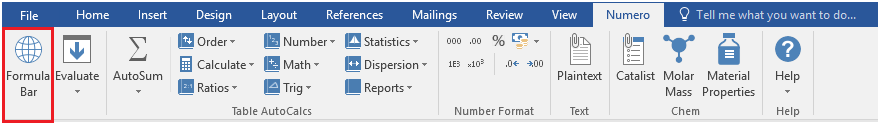This will open Formula Bar, which consists of an Input box, an arrow that points to a dropdown menu that will contain the result(s) from your input, as shown: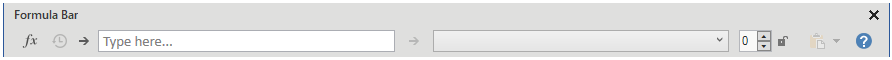The box to the right-hand side of this dropdown menu, is for selecting the number of decimal places that you want your output shown in. The maximum decimal places is 15. The lock symbol to the right of this Lock in the amount of decimal places.  When this is open, you can change the number of decimal places, however, when it is closed, it cannot be modified.

The next icon to the right of the lock icon is for pasting the result(s) into the document. When you click on the dropdown menu, you should see 3 options : Paste, Paste to Plaintext and Copy, as shown below. The Paste option pastes the result into the document you are working on, the Paste to Plaintext option opens Plaintext and pastes the result there and the copy option copies the result onto the clipboard.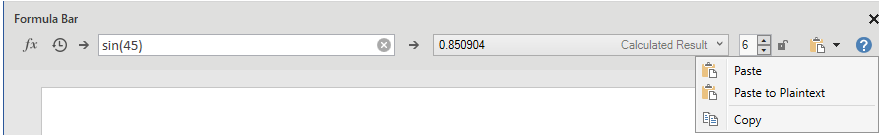There are 3 icons to the left of the input box as shown below: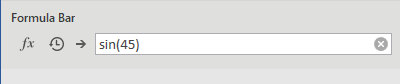The fx icon inserts a function. For example, if you click on that icon, a list of functions will appear. Double clicking on one of these will insert the function.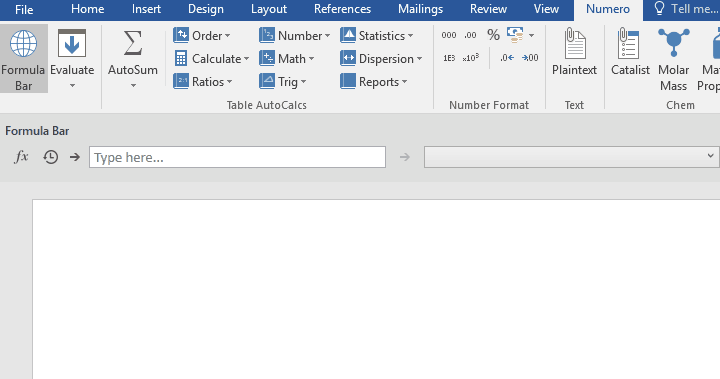The other two icons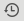shows recently used formulas when selected and the icon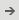Loads the Selection. This is most useful when you select the contents from table of numbers and click on this icon. This will load the number into the input box.

Formula calculations

Formula Bar can used to directly enter a calculation such as SIN(45) or ROUND(3.1456; 2) if you want to want to round the number to 2 digits.

If you have an array of numbers, input these numbers, you can input these numbers directly into formula bar. For example, if you want to calculate the mean of 1, 2, 3, 4, 5 and 6, you would input them in the following format: MEAN({1;2;3;4;5;6}), the key here is that your array of numbers must be enclosed in braces (curly brackets. These 3 calculations are shown below: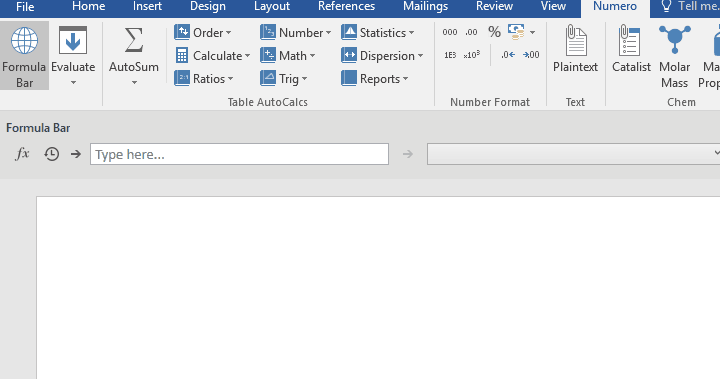You can also search for the function using the fx icon as shown below: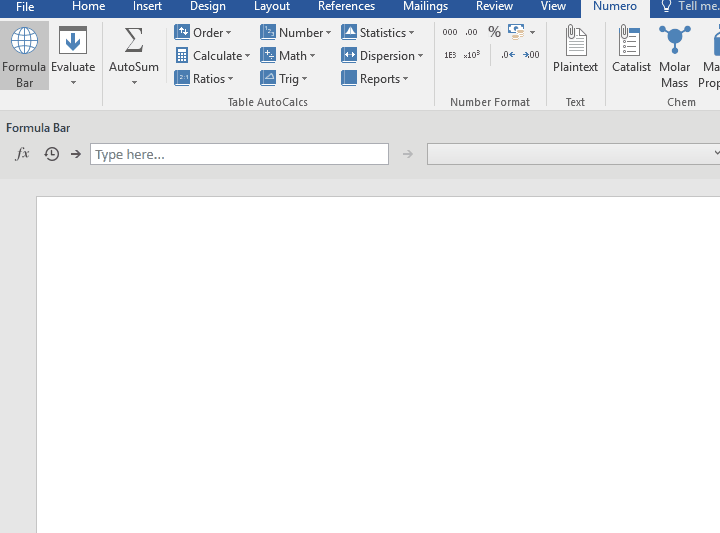If you have a table in your document containing a random set of numbers, you can Load these numbers into Formula Bar by clicking in the arrow icon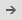and then selecting the formula you want to use. See below for an example of how to calculate the SUM of a table of numbers and pasting the value in your Word document.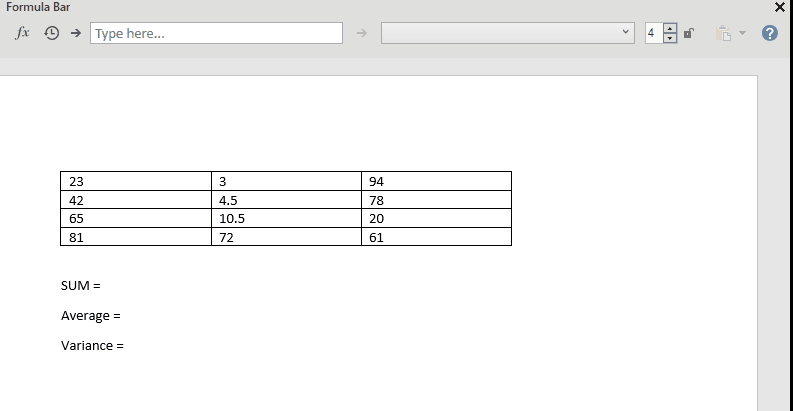After loading a table of numbers, you will see the following icon on the right of the input box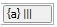. If you hover over that icon, you will see a tooltip with your data in a table and that your table was either loaded in columns or rows where the symbol |  ||  ||| indicates one, two or three columns respectively. Similarly,  if you see one, two or three horizontal lines as shown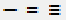, this means that the data is loaded in rows.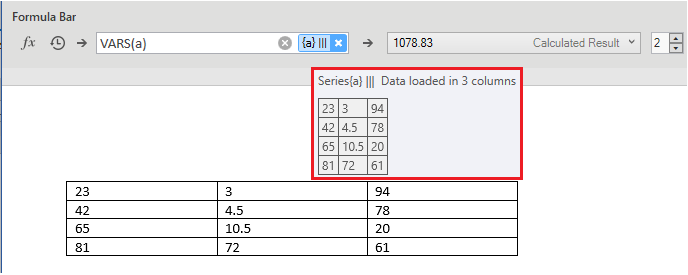If your table is loaded in column and you want to change that to rows, right-clicking on thewill bring you to a the menu below where you can select to Load as Rows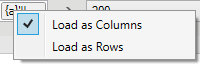Currency converter

If you want to convert a currency to one other currency using Formula Bar, then type in the currency that you want to convert from, followed by the amount followed by the currency that you want to convert to. For example if you want to convert 50 US dollars into Euro, you would type in USD 50 EUR.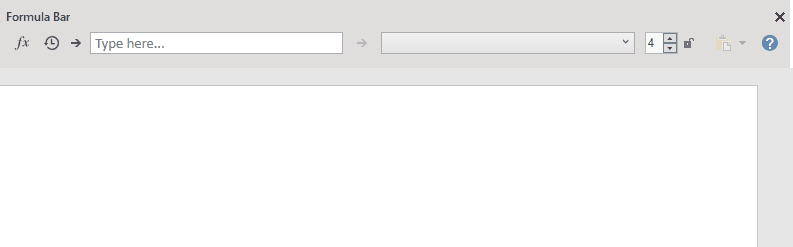If you want to convert 50 US dollars to more than ones currency such as Euro, British pounds and Indian Rupees, then you type in the currency that you want to convert from, the amount and then the currencies that you want to convert to. This is shown below: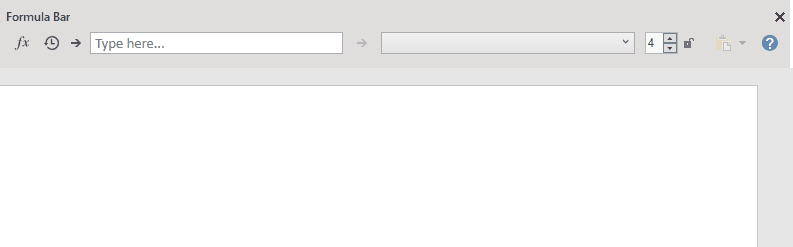If you have typed USD followed by the amount (e.g. 50), then pressed enter, you will see the currency converted into nine other currencies, as shown below: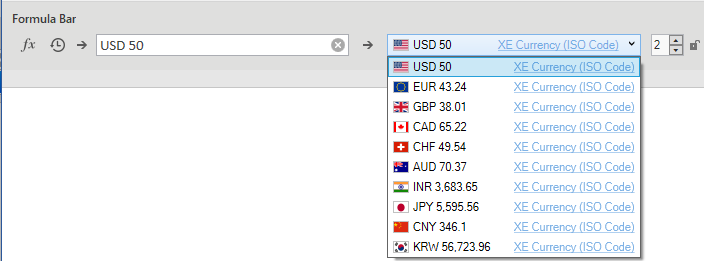You can also input the currency symbols in formula bar, for example \$ 10 £ and you will see the following conversion when you click on the dropdown menu: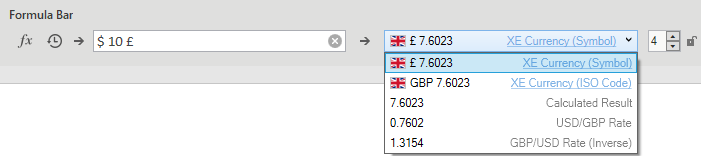You can also carry out the currency conversion from your working document. For example, if you have USD 50 and you Load it into Formula Bar, then you will see 50 USD in the input box and the relevant conversions in the output box. This is shown below: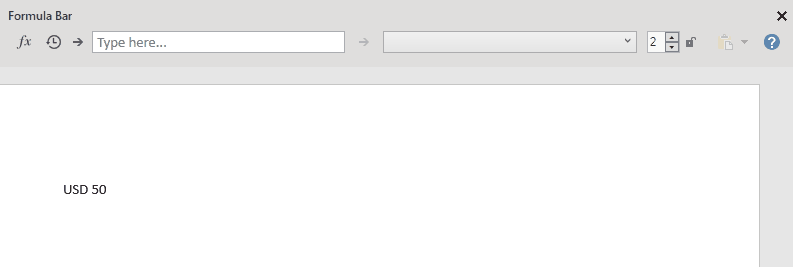Alternatively, if you want you convert 50 USD into British pound, you can type in USD 50 GBP, select this, and Load it into Formula Bar. From there, you will see the conversion of USD into GBP in the output box.

A final way that you can use currency conversion is by using the either typing in XE into Formula Bar or by clicking on the fx icon and selecting XE(x; from; to) if you have a single value that you want to convert, or XE(a; from; to) if you have an array (table) of values that you want to convert. Both of these are shown below: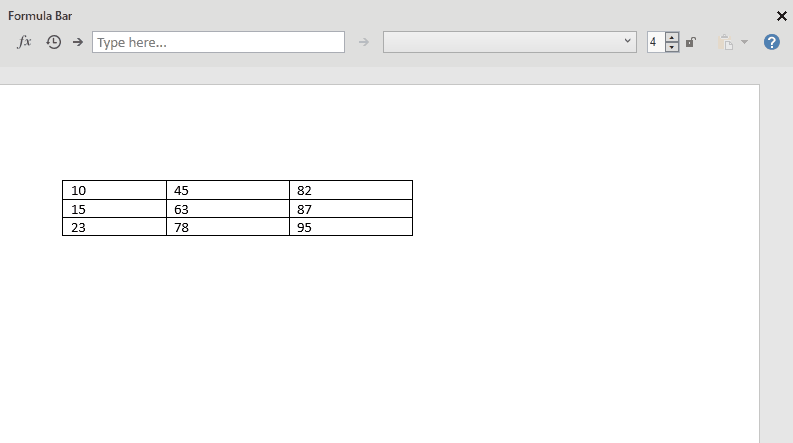Note: If you want to clear the content of the input box, click on the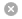icon.

Unit converter

How it works?

Units available

 Length Luminous Flux Radiant intensity Mass Illuminance Radiance Time Catalytic activity Catalytic activity concentration Electric current Absorbed dose Concentration Temperature Dose equivalent Absorbed dose rate Amount of substance Dynamic viscosity Thermal diffusivity Luminous intensity Solid angle Thermal insulance Area Angle Thermal resistivity Volume Moment of force Density Velocity Surface tension Kinematic viscosity Acceleration Angular velocity Volumetric flow rate Force Frequency Molar mass Pressure Angular acceleration Density of heat Energy Heat flux density Mass flow rate Power Heat capacity Active site per unit volume Electric charge Specific heat capacity Specific conductance Capacitance Thermal conductivity Cubical expansion coefficient Electric resistance Energy density Henrys law coefficients Electric conductance Electric field strength Electric dipole moment Voltage Electric charge density Specific volume (molar) Frequency Permittivity van der Waals area Activity Permeability Coefficient of heat transfer Magnetic flux Molar energy Thermal conductance Inductance Molar heat capacity Sound intensity Magnetic Flux Density Exposure